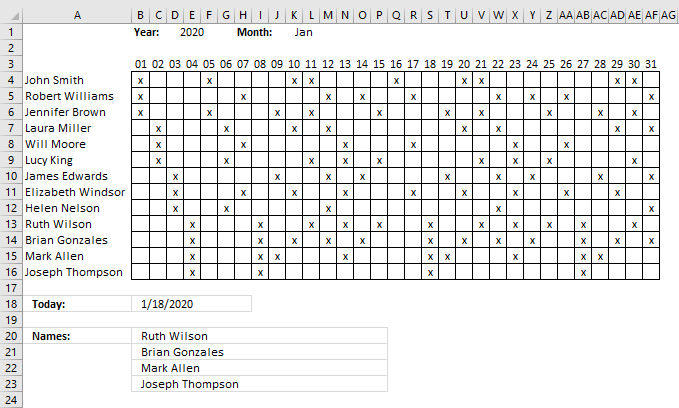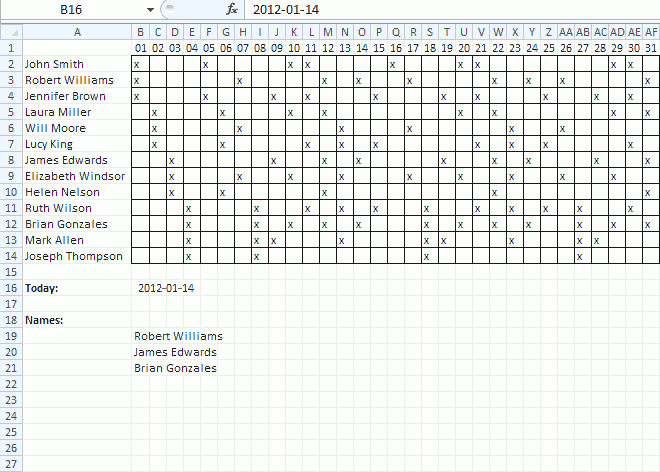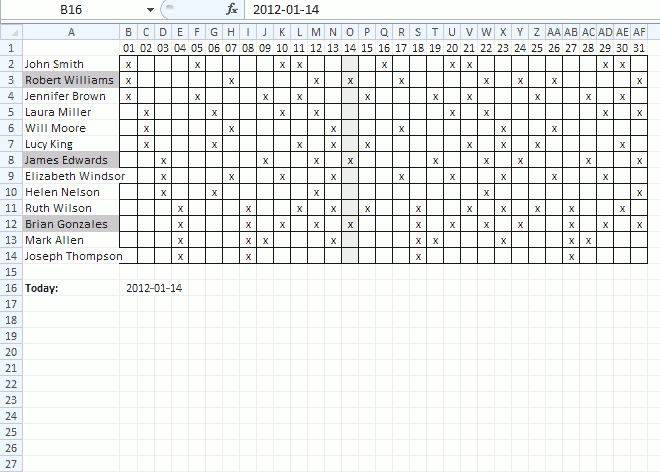Author: Oscar Cronquist Article last updated on April 10, 2019Hi Oscar, I have a cross reference table we use for shift scheduling. The x-axis is comprised of dates and the y is names. The table values indicate whether or not the employee is scheduled to work (i.e., filled or not).

Is there any way to pull a list of names in a canned daily report based on the date and whether the cell is filled? In other words, I want to look up the y-axis headers as opposed to the cross reference value.

Thanks, Geoff

The image above demonstrates a schedule with dates on row 3, they are calculated automatically based on the selected year in cell D1 and month in K1.

You have to enter the names in column A and if they are scheduled for work (filled x) in the corresponding cells. Cell B18 returns the current date today and cell B20 and below return the names of the people that work that particular day.

Formula in cell B18:

=TODAY()

Array formula in cell B20:

=IFERROR(INDEX(\$A\$4:\$A\$16,SMALL(IF(INDEX(\$B\$4:\$AF\$16,0,MATCH(\$B\$18,\$B\$3:\$AF\$3,0))="x",MATCH(ROW(\$A\$4:\$A\$16),ROW(\$A\$4:\$A\$16)),""),ROWS(\$A\$1:A1))),"")

#### How to create an array formula

1. Select cell B19
2. Paste array formula in formula bar3. Press and hold CTRL + SHIFT
4. Press Enter

#### How to copy array formula

1. Select cell B20
2. Copy (Ctrl + c)
3. Select cell range B21:B26
4. Paste (Ctrl + v)

### Explaining array formula in cell B20#### Step 1 - Find position of current date on row 3

This so we can extract the entire column in a later step. The MATCH function allows us to identify the position of a given value in an array or cell range.

The cell range must, however, have only one column or row. In other words, the cells must be horizontally or vertically arranged.

MATCH(\$B\$18, \$B\$3:\$AF\$3, 0)

becomes

MATCH(40926, {40909, 40910, 40911, 40912, 40913, 40914, 40915, 40916, 40917, 40918, 40919, 40920, 40921, 40922, 40923, 40924, 40925, 40926, 40927, 40928, 40929, 40930, 40931, 40932, 40933, 40934, 40935, 40936, 40937, 40938, 40939}, 0)

and returns 18.

Excel date 40926 is found in the 18th position of cell range \$B\$1:\$AF\$1.

#### Step 2 - Extract values in a specific column

The INDEX function lets you extract a specific column based on a column number and a cell range to an array.

INDEX(cell_ref, row_num, [column_num], [area_num]

INDEX(\$B\$4:\$AF\$14, 0, MATCH(\$B\$18, \$B\$3:\$AF\$3, 0))

becomes

INDEX(\$B\$4:\$AF\$14, 0, 18)

and returns the values in cell range S2:S14.

{0; 0; 0; 0; 0; 0; 0; 0; 0; "x"; "x"; "x"; "x"}

#### Step 3 - Identify which cells contain x

The equal sign compares each value in the array with x, if they match TRUe is returned and FALSE if not. TRUE and FALSE are boolean values.

INDEX(\$B\$4:\$AF\$14, 0, MATCH(\$B\$18, \$B\$3:\$AF\$3, 0))="x"

becomes

{0; 0; 0; 0; 0; 0; 0; 0; 0; "x"; "x"; "x"; "x"}="x"

and returns

{FALSE; FALSE; FALSE; FALSE; FALSE; FALSE; FALSE; FALSE; FALSE; TRUE; TRUE; TRUE; TRUE}

#### Step 4 - Replace TRUE with corresponding row number and FALSE with blanks

The IF function returns a value if the logical expression is TRUE and another value if FALSE. Since we are working with arrays the values returned are determined by the position of the value in the array.

IF(INDEX(\$B\$4:\$AF\$14, 0, MATCH(\$B\$18, \$B\$3:\$AF\$3, 0))="x", MATCH(ROW(\$A\$4:\$A\$16), ROW(\$A\$4:\$A\$16)), "")

becomes

IF({FALSE; FALSE; FALSE; FALSE; FALSE; FALSE; FALSE; FALSE; FALSE; TRUE; TRUE; TRUE; TRUE}, MATCH(ROW(\$A\$4:\$A\$16), ROW(\$A\$4:\$A\$16)), "")

becomes

IF({FALSE; FALSE; FALSE; FALSE; FALSE; FALSE; FALSE; FALSE; FALSE; TRUE; TRUE; TRUE; TRUE}, {1;2;3;4;5;6;7;8;9;10;11;12;13}, "")

and returns

{""; ""; ""; ""; ""; ""; ""; ""; ""; 10; 11; 12; 13}.

#### Step 5 - Extract the k-th smallest row number

The SMALL function allows you to extract a single value in each cell based on a row number.

SMALL(IF(INDEX(\$B\$4:\$AF\$14, 0, MATCH(\$B\$18, \$B\$3:\$AF\$3, 0))="x", MATCH(ROW(\$A\$4:\$A\$16), ROW(\$A\$4:\$A\$16)), ""), ROWS(\$A\$1:A1))

becomes

SMALL({""; ""; ""; ""; ""; ""; ""; ""; ""; 10; 11; 12; 13}, ROWS(\$A\$1:A1))

The ROWS function has a cell reference that changes when you copy the cell and paste to cells below, this is what makes the formula extract a new value in each cell.

SMALL({""; ""; ""; ""; ""; ""; ""; ""; ""; 10; 11; 12; 13},1)

and returns 10.

#### Step 6 - Get the name from column A based on a row number

The INDEX function lets you extract a value based on a cell range and a row and column number. Our cell range contains only one column so we can leave out the column number.

INDEX(\$A\$4:\$A\$16, SMALL(IF(INDEX(\$B\$4:\$AF\$14, 0, MATCH(\$B\$18, \$B\$3:\$AF\$3, 0))="x", MATCH(ROW(\$A\$4:\$A\$16), ROW(\$A\$4:\$A\$16)), ""), ROWS(\$A\$1:A1)))

#### Step 7 - Return blank if formula returns an error

The IFERROR function handles all errors so be careful if you use this function. If there is something else wrong you won't spot it because the IFERROR function returns a blank in that case as well.

IFERROR(INDEX(\$A\$4:\$A\$16, SMALL(IF(INDEX(\$B\$4:\$AF\$14, 0, MATCH(\$B\$18, \$B\$3:\$AF\$3, 0))="x", MATCH(ROW(\$A\$4:\$A\$16), ROW(\$A\$4:\$A\$16)), ""), ROWS(\$A\$1:A1))), "")### Get the Excel fileShift-schedulingv3.xlsx

### Shift schedule - Conditional formatting### Conditional formatting - Cell range B2:AF14

1. Select cell range B2:AF14
2. Go to tab "Home"
3. Press with left mouse button on "Conditional formatting" button
4. Press with left mouse button on "New Rule.."
5. Press with left mouse button on "Use a formula to determine which cells to format"
6. Enter this formula:=B\$1=\$B\$16
7. Press with left mouse button on "Format.." button
8. Go to tab "Fill"
9. Pick a color
10. Press with left mouse button on OK
11. Press with left mouse button on OK

### Conditional formatting - Cell range A2:A14

1. Select cell range A2:A14
2. Go to tab "Home"
3. Press with left mouse button on "Conditional formatting" button
4. Press with left mouse button on "New Rule.."
5. Press with left mouse button on "Use a formula to determine which cells to format"
6. Enter this formula:=INDEX(\$B\$2:\$AF\$14, ROW(A2)-1, MATCH(\$B\$16, \$B\$1:\$AF\$1, 0))="x"
7. Press with left mouse button on "Format.." button
8. Go to tab "Fill"
9. Pick a color
10. Press with left mouse button on OK
11. Press with left mouse button on OK